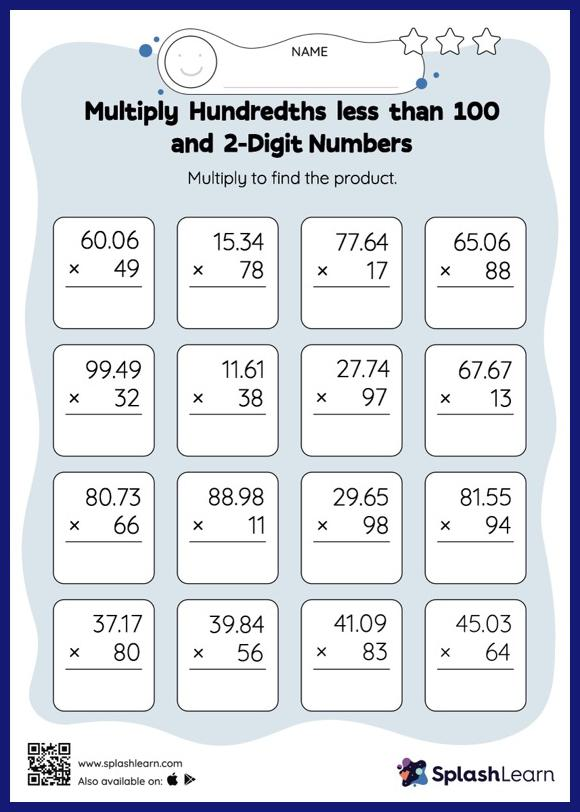# Multiply Hundredths less than 100 and 2-Digit Numbers: Vertical Multiplication Worksheet

Home > Multiply Hundredths less than 100 and 2-Digit Numbers: Vertical MultiplicationChildren use their math skills to complete this multiply hundredths less than 100 and 2-digit numbers worksheet. Students may omit the decimal point when multiplying a decimal by a two-digit number and then add it when writing the answer. This multiply hundredths less than 100 and 2-digit numbers worksheet gives learners many opportunities to practice this concept.